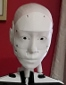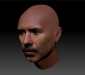# version 4 of robot legs

I have continued to work on the robot legs and have them 'static' walking

All the main support parts are made in aluminum

Still using RoboClaw boards for driving the 10 motors, all motors have encoders

New all joints have angle sensors

I have a IMU on the platform and am using roll and pitch

power is from 3 LION 3.7 volt cells on the top back,  cells are from a hybrid car

I finally listened to Ray and have the weight on the top, inverted pendulum

A raspberry pi is mounted on the electronics panel and I hope to use it to make decisions on what to do.

Currently the Arduino Mega is running everything.  The Pi should enable me to use MRL.

you tube https://youtu.be/rR0FFqIo8PE

since I machined a lot of alum. parts, I wouldn't expect othe people to make these.

The ABS parts in my eariler legs didn't take the stress

I am collecting data from the IMU, angle senstor, roboclaw boards  example:

Leg version: Dec  8 2021 19:28:42   took 3 steps on batteries

number of steps to take=3

Step=1 ** RIGHT LEG

* Encd                        if the value is 0 encoders don't show any value

* Ang                         if the angle sensor is at 0 it does not show any value

* IMU, Roll=5, Pitch=-5, Yaw=129, Heading=214, Lps=0, StrLen=31

- Right Ankle Roll out left ankle roll in legs lean to the right

* Speed RgtAnkRol=-411 LAnkRot=-199

* Amp, RAkR=0.5, LAkP=0.0, LAkR=0.2, TAmp=0.7

# No Cmds in Buffer,

# Loops waiting (200ms/each)=1

* Encd

* Ang, LHipPt=3, RKnePt=-1, LKnePt=-2, LAnkPt=-3, RAnkPt=-2

* IMU, Roll=0, Pitch=1, Yaw=128, Heading=216, Lps=0, StrLen=30

* IMU, Roll=16, Pitch=3, Yaw=130, Heading=223, Lps=0, StrLen=31

- left knee bends up and left foot lifts up

- right foot-ankle bends up shifting body forward

- right hip rolls back, moving electronics toward center

- left leg move up toward hip, making leg go out, falls forward

* Speed RghHipPit=-793 LKnePit=-3037 LHipPit=328 RgtAnkPit=21283 LAnkPit=14895

VLowBbd1=11.4VLowBbd2=11.4VLowBbd3=11.4VLowBbd4=11.4VLowBbd5=11.4

* Amp, RKnP=0.1, RHpP=0.6, LHpP=0.9, LKnP=3.0, RAkP=1.9, LAkP=2.8, LAkR=0.2, TAmp=9.5

# CmdBuf=129,0,0# CmdBuf=130,0,0# CmdBuf=131,0,0# CmdBuf=132,0,0# CmdBuf=133,0,0

* Speed RghHipPit=-423 LKnePit=-154 LHipPit=43 RgtAnkPit=1566 LAnkPit=903

* Amp, RKnP=0.1, RHpP=0.4, LKnP=0.2, RAkP=1.3, LAkP=1.7, LAkR=0.2, TAmp=3.9

# CmdBuf=129,0,0# CmdBuf=130,0,0# CmdBuf=131,0,0# CmdBuf=132,0,0# CmdBuf=133,0,0

* Speed RghHipPit=-35 LKnePit=-7 LHipPit=2 RgtAnkPit=72 LAnkPit=41

* Amp, RKnP=0.1, RHpP=0.2, LKnP=0.2, RAkP=0.2, LAkP=0.2, LAkR=0.2, TAmp=1.1

# No Cmds in Buffer,

# Loops waiting (200ms/each)=3

* Encd

* Ang, LHipPt=-6, RHipPt=-5, RKnePt=-1, LKnePt=12, LAnkPt=-1

* IMU, Roll=7, Pitch=1, Yaw=131, Heading=231, Lps=0, StrLen=30

- left knee straight, left ankle rotate back to 0, left foot flat

* Speed LKnePit=6 LAnkRot=20

* Amp, RKnP=0.1, LKnP=2.4, RAkP=0.2, LAkR=0.0, TAmp=2.7

# No Cmds in Buffer,

# Loops waiting (200ms/each)=1

* Encd

* Ang, LHipPt=-5, RHipPt=-5, RKnePt=-1, LKnePt=-2, LAnkPt=-3

* IMU, Roll=9, Pitch=3, Yaw=136, Heading=230, Lps=0, StrLen=30

- right ankle rotate back to 0

* Speed LKnePit=1 RgtAnkRol=1

* Amp, RKnP=0.1, LKnP=2.4, RAkP=0.2, TAmp=2.7

# CmdBuf=129,0,0# CmdBuf=130,0,0# CmdBuf=131,0,0# CmdBuf=132,0,0# CmdBuf=133,0,0

# Loops waiting (200ms/each)=1

* Encd, RHipP=-1331, RAnkR=2, RAnkP=30260, LKneP=-1, LHipP=1176, LAnkR=-11, LAnkP=-8

* Ang, LHipPt=-8, RHipPt=-5, RKnePt=-353, LAnkPt=-2

* IMU, Roll=9, Pitch=2, Yaw=135, Heading=229, Lps=0, StrLen=30

- feet flat, left & right hip to 0

* Speed RgtAnkPit=529

* Amp, RKnP=0.0, RHpP=0.5, LHpP=1.2, LKnP=2.5, RAkP=1.3, TAmp=5.5

# No Cmds in Buffer,

# Loops waiting (200ms/each)=1

* Encd

* Ang, LHipPt=-9, RHipPt=-2, LAnkPt=-3, RAnkPt=2

* IMU, Roll=-1, Pitch=-4, Yaw=131, Heading=211, Lps=0, StrLen=32

** LEFT LEG

- right and left ankle rotate left legs leans to the left

* Speed RgtAnkRol=527 RgtAnkPit=35 LAnkRot=593

* Amp, RKnP=0.1, RHpP=0.4, LHpP=1.3, LKnP=2.7, RAkP=0.9, RAkR=0.3, LAkR=0.3, TAmp=6.0

# CmdBuf=129,0,0# CmdBuf=130,0,0# CmdBuf=131,0,0# CmdBuf=132,0,0# CmdBuf=133,0,0

* Speed RgtAnkRol=45 RgtAnkPit=13 LAnkRot=145

* Amp, RKnP=0.0, RHpP=0.4, LHpP=1.3, LKnP=2.6, RAkP=0.9, TAmp=5.3

# CmdBuf=129,0,0# CmdBuf=130,0,0

* Speed RgtAnkRol=2 RgtAnkPit=6 LAnkRot=8

* Amp, RKnP=0.0, RHpP=0.4, LHpP=1.2, LKnP=2.6, RAkP=0.2, TAmp=4.5

# CmdBuf=129,0,0# CmdBuf=130,0,0

# Loops waiting (200ms/each)=3

* Encd, RAnkR=1026, RAnkP=-15, LKneP=1, LHipP=9, LAnkR=998, LAnkP=-8

* Ang, LHipPt=-9, RHipPt=-2, RKnePt=-353, LAnkPt=-1, RAnkPt=2

* IMU, Roll=-1, Pitch=-3, Yaw=129, Heading=212, Lps=0, StrLen=32

* IMU, Roll=-6, Pitch=-3, Yaw=128, Heading=205, Lps=0, StrLen=32

roll loops=1

* Speed RghHipPit=-51 LHipPit=-4

* Amp, RKnP=0.1, RHpP=0.1, LHpP=0.4, LKnP=2.5, RAkP=1.6, TAmp=4.7

# CmdBuf=129,0,0# CmdBuf=130,0,0# CmdBuf=131,0,0# CmdBuf=132,0,0# CmdBuf=133,0,0

* Speed RghHipPit=-37

* Amp, RKnP=0.1, RHpP=0.2, LKnP=2.4, RAkP=1.7, TAmp=4.4

# CmdBuf=129,0,0# CmdBuf=130,0,0

# Loops waiting (200ms/each)=2

* Encd, RHipP=-18, RAnkR=1183, RAnkP=-12, LKneP=1, LHipP=-28, LAnkR=1169, LAnkP=-8

* Ang, LHipPt=-8, RKnePt=-352, LAnkPt=-1, RAnkPt=3

* IMU, Roll=-7, Pitch=-7, Yaw=126, Heading=205, Lps=0, StrLen=32

* IMU, Roll=-9, Pitch=-5, Yaw=125, Heading=203, Lps=0, StrLen=32

- right knee bends up and right foot bends up, lifting leg

- left foot-ankle bends up shifting body forward

- left hip rolls back, moving electronics toward center

- right leg move up toward hip, making leg go out, legs tip forward

* Speed RghKnePit=-444 RghHipPit=583 LHipPit=-716 RgtAnkPit=15793 LAnkPit=25

VLowBbd1=11.4VLowBbd2=11.4* Amp, RHpP=0.2, LHpP=1.1, LKnP=2.6, RAkP=1.0, LAkP=3.8, TAmp=8.8

# CmdBuf=129,0,0# CmdBuf=130,0,0# CmdBuf=131,0,0# CmdBuf=132,0,0# CmdBuf=133,0,0

* Speed RghKnePit=-20 RghHipPit=62 LHipPit=-689 RgtAnkPit=1275 LAnkPit=7

* Amp, RHpP=0.2, LHpP=1.3, LKnP=2.9, RAkP=0.2, LAkP=4.0, TAmp=8.5

# CmdBuf=129,0,0# CmdBuf=130,0,0# CmdBuf=131,0,0# CmdBuf=132,0,0# CmdBuf=133,0,0

* Speed RghKnePit=-1 RghHipPit=3 LHipPit=-662 RgtAnkPit=67

* Amp, LHpP=1.3, LKnP=2.7, RAkP=0.2, LAkP=3.8, TAmp=8.0

# CmdBuf=129,0,0# CmdBuf=130,0,0# CmdBuf=131,0,0# CmdBuf=132,0,0# CmdBuf=133,0,0

* Speed LHipPit=-654 RgtAnkPit=3 LAnkPit=9

* Amp, LHpP=1.3, LKnP=2.6, RAkP=0.2, LAkP=4.1, TAmp=8.2

# CmdBuf=129,0,0# CmdBuf=130,0,0# CmdBuf=131,0,0# CmdBuf=132,0,0# CmdBuf=133,0,0

* Speed LHipPit=-324 LAnkPit=4

* Amp, LHpP=1.1, LKnP=2.6, RAkP=0.2, LAkP=4.0, TAmp=7.8

# CmdBuf=129,0,0# CmdBuf=130,0,0# CmdBuf=131,0,0# CmdBuf=132,0,0# CmdBuf=133,0,0

* Speed LHipPit=-27

* Amp, LHpP=0.9, LKnP=2.7, RAkP=0.2, LAkP=4.0, TAmp=7.8

# CmdBuf=129,0,0# CmdBuf=130,0,0# CmdBuf=131,0,0# CmdBuf=132,0,0# CmdBuf=133,0,0

# Loops waiting (200ms/each)=6

* Encd, RKneP=-21058, RHipP=1465, RAnkR=1183, RAnkP=41289, LKneP=1, LHipP=-1712, LAnkR=1169, LAnkP=1709

* Ang, LHipPt=4, RHipPt=3, RKnePt=-11, LAnkPt=-1, RAnkPt=1, LHipYw=1, RHipYw=5

* IMU, Roll=-7, Pitch=-6, Yaw=125, Heading=203, Lps=0, StrLen=32

- right foot flat(pitch), right knee straight(pitch)

- right & left ankles roll flat

* Speed RghKnePit=866 LKnePit=2 RgtAnkRol=6 LAnkPit=123

VLowBbd1=10.9VLowBbd2=10.9VLowBbd3=10.9VLowBbd4=10.9VLowBbd5=10.9

* Amp, RKnP=2.6, LKnP=2.5, RAkP=0.8, LAkP=3.9, TAmp=9.8

# CmdBuf=133,128,0

* Speed RghKnePit=59 LAnkPit=77

* Amp, RKnP=2.3, LKnP=2.4, RAkP=0.9, LAkP=4.0, TAmp=9.6

# CmdBuf=129,0,0# CmdBuf=130,0,0# CmdBuf=131,0,0# CmdBuf=132,0,0# CmdBuf=133,0,0

* Speed RghKnePit=3 LAnkPit=9

* Amp, RKnP=0.6, LKnP=2.3, RAkP=0.9, LAkP=4.1, TAmp=8.0

# CmdBuf=129,0,0# CmdBuf=130,0,0# CmdBuf=133,128,0

# Loops waiting (200ms/each)=3

* Encd, RKneP=14, RHipP=1465, RAnkR=10, RAnkP=-2, LKneP=9, LHipP=-1712, LAnkR=5, LAnkP=3517

* Ang, LHipPt=4, RHipPt=3, RKnePt=-1, LKnePt=-2, RAnkPt=-1

* IMU, Roll=-8, Pitch=5, Yaw=127, Heading=213, Lps=0, StrLen=31

- left foot flat(pitch), both hips to 0(pitch)

* Speed RghHipPit=-44

* Amp, RKnP=7.0, RHpP=1.9, LKnP=0.2, RAkP=0.9, TAmp=10.0

# CmdBuf=129,0,0

# Loops waiting (200ms/each)=1

* Encd, RKneP=13, RHipP=-9, RAnkR=10, RAnkP=9, LKneP=12, LHipP=26, LAnkR=5, LAnkP=-32

* Ang, LHipPt=3, RHipPt=3, RKnePt=-1, RAnkPt=1

* IMU, Roll=5, Pitch=0, Yaw=131, Heading=219, Lps=0, StrLen=30

* Encd, RKneP=11, RHipP=-14, RAnkR=10, RAnkP=9, LKneP=12, LHipP=26, LAnkR=5, LAnkP=-32

violet above is encoders at end on first step

* Ang, LHipPt=3, RHipPt=3, RKnePt=-1, RAnkPt=1

* IMU, Roll=0, Pitch=6, Yaw=129, Heading=219, Lps=0, StrLen=30

-- done### Making Progress.

Having that mass up there should also give more confidence that it will carry the Inmoov robot on top of the legs.

It's good to see your still working on it :-)

Ray### Wahoo, John, nice to see your

Wahoo, John, nice to see your progressing on the legs!

You may want to join the mrl discord chanel, it would be nice to have you around again.